Maths-
General
Easy

Question

# a =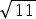+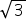, b =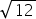+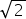and c =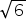+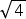, then which of the following holds true?i) c > a > b     ii) a > b > c    iii) a > c > b     iv) b > a > cHint:

## The correct answer is: a > b > c

### Step 1 of 2:              a =+Squaring both sides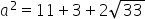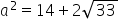.......  (1)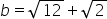Squaring both sides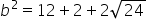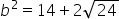..... (2)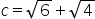Squaring both sides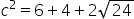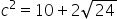Step 2 of 2:We can see that from equation (1) and (2), a2 > b2 which means a > b, and from equation (2) and (3) b2 > c2 which means b > c.Final Answer: We found that a > b and b > c. So, we can conclude that a > b > c. Hence, option (ii) is correct.

Note:
This question can simply be solved by finding the values of all the square roots and then using them in finding the values of a, b and c and then their values can be easily compared.#### With Turito Foundation.#### Get an Expert Advice From Turito.